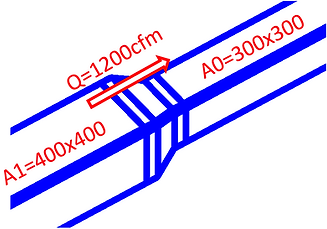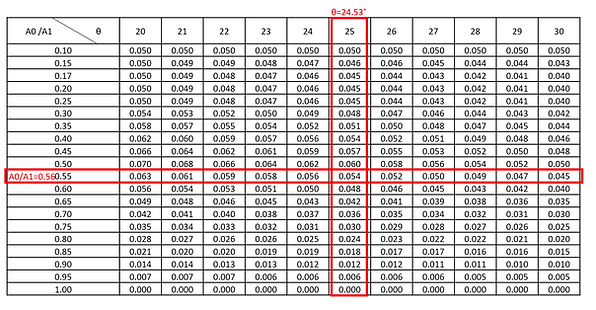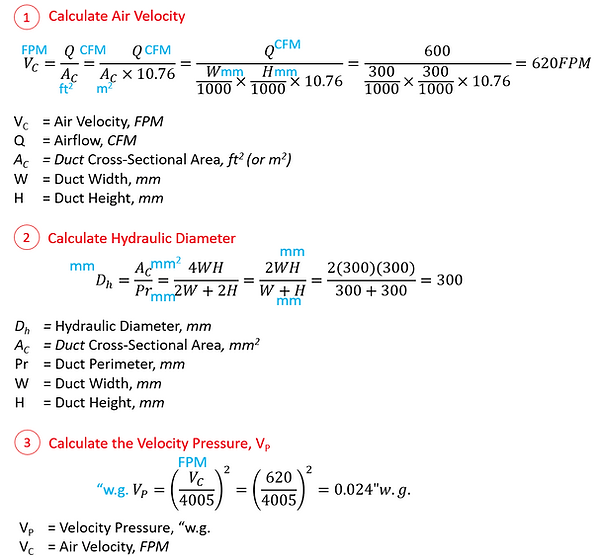top of page
Summary Table

How to Compute External Static Pressure Drop (ESP) for a Duct System

The following explains the simple steps to compute pressure drop according to the Ashrae standard.

A simple example of duct network sized based on the equal friction method is as shown below.First, we determine the critical path of duct along which there is the highest pressure loss. Bear in mind that this path does not necessarily be a path with the longest route, but the loss largely subject to the airflow, Q (CFM) within the path of duct and fittings.

Then, a tabulation is established to determine the pressure drop at each of the sections of the duct network, labeled at the nodes, which may comprise of the duct run as well as the duct fittings.The Excel file attached below is an example of how pressure drop can be tabulated and computed. It consists of different tabs for the determination of duct and fitting losses at different nodes. Below we will pick a few example to show how these losses are generally being computed.

Node A

For this straight length of duct, Refer to . The 6 main steps are being eloborated with the example at Node A below:Node B

For this fire damper, Refer to How to Compute Pressure Drop across a Fire Damper. Either the general formula below can be applicable, a suitable Ashrae fitting can be referred to, or the pressure drop data from a manufacturer’s guideline may be a suitable reference.Node C

To determine the loss for a transition, Refer to How to Compute Pressure Drop across a Duct Transition.

According to the Ashrae fitting (SR4-2), the transition loss is based on a few parameters, namely

1. The length of transition, L

2. Pre-transition duct Width, W & Height, H (Contributes to Area A1)

3. Post-transition duct Width, W & Height, H (Contributes to Area A0)

4. Airflow, QBelow are the steps for the calculation of the losses based on a simple example above:

1. From W, H, and L, the angle θ for both the sides and and the top (θ1 and θ2) can be computed as shown below. Take the bigger value between the 2 angles (θ1 and θ2) as θ, i.e.

θ = MAX(θ1, θ2) = 24.53 deg2. Then, compute the ratio of Duct Cross Sectional Area,

A0/A1 = (300x300)/(400x400) = 0.753. From the Ashrae table, determine the Coefficient of Loss, C can be determined with information computed from Step 1 and 2 above.Where no exact value of theta and A0/A1 can be referenced in the fitting loss table, Interpolation using “Forecast” function in excel may be helpful to determine the Coefficient of Loss. From the interpolated data shown below, we see that the coefficient of loss, C = 0.054.4. Compute the Velocity Pressure, VP post-transition (for exhaust, pre-transition)

5. Compute Pressure Loss, ΔP byNode G

For smooth elbows without vane, refer to How to Compute Pressure Drop across a Duct Elbow.

Below are the steps for the calculation of the losses:Again, where no exact value of r/W and H/W can be referenced in the fitting loss table, Interpolation using “Forecast” function in excel may be helpful to determine the Coefficient of Loss.

The total external static pressure required by the fan shall be the sum of the pressure drop across all nodes along the critical path as tabulated in the summary above, including those not shown in the 4 examples shown above (Node A, B, C and G).bottom of page